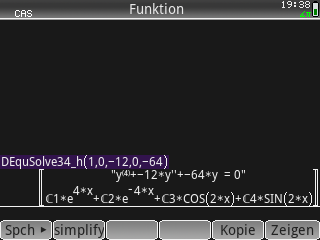## Differential Equation of 4th and 3rd Order, Part 2

Description:Finds the solution y(x) = y_hom + y_part to the differential equation (D.E.) of 4th order a*y^(4) + b*y''' + c*y'' + d*y' + e*y = g(x) or of 3rd order (a = 0) b*y''' + c*y'' + d*y' + e*y = g(x), where g(x) = e^(alpha*x) * [c1*SIN(beta*x) + c2*COS(beta*x)].
Filename:diffequat34part2.zip
ID:9232
Current version:1.02
Author:Claus Martin Dachselt
Size on calculator:10 KB
Platforms:Prime
User rating:Not yet rated (you must be logged in to vote)
Primary category:Math
Languages:ENG
File date:2020-12-02 08:32:41
Creation date:2020-12-02
Source code:Not included
Related files:Differential Equation of 4th and 3rd Order, Part 1
Screenshot: